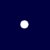# Animated Graphical Dice

We have shown how to create a dice in our example, Graphical Dice. However, we need to refresh the web page in order to change the faces of the dice, therefore, it is not suitable for playing games.

In this example, we will create an animated dice that changes rapidly when we click the roll dice button. In order to create an animated dice, first of all, you need to design 6 images of a dice that represent  1, 2, 3,4, 5 and 6, Next, we need to create a JavaScript function that can generate an animated dice.

To create animation, we use the setInterval method, the syntax is as follows:

`myVariable=setInterval(myfunction, time interval)`

The setInterval method runs a function created by you at a certain time interval in milliseconds.  For example,  a time interval of 1000 equals to 1 second.

We use the following syntax to create 6 random numbers:

`ranNum = Math.floor( 1 + Math.random() * 6 );`

We also use the following statement to load the first image as default image.

`<img id="die" src="1.jpg" width="50" height="50">`

The id is important as we shall use it to load other images when animation starts, as shown in the following code:

```var ranNum = Math.floor( 1 + Math.random() * 6 );
var dice = document.getElementById("die");
dice.src=ranNum+".jpg";
}
```

ranNum will be a random integers from 1 to 6, and by using the src property and combining ranNum+".jpg", we will be able to load the 6 images randomly.

##### The JavaScript
```<html>
<meta http-equiv="Content-Type" content="text/html; charset=windows-1252">
<title>JavaScript Graphical Dice Function</title>
<meta name=viewport content="width=device-width, initial-scale=1">

<body>
<img id="die" src="1.jpg" width="50" height="50">

<button onclick="AniDice()">Roll Dice</button>
<button onclick="stopDice()">Stop</button>

<script>

function AniDice()
{
myVar=setInterval(rolldice,20)
}

function rolldice()
{
var ranNum = Math.floor( 1 + Math.random() * 6 );
var dice = document.getElementById("die");
dice.src=ranNum+".jpg";
}
function stopDice()
{clearInterval(myVar);}
</script>

</body>
</html>
```